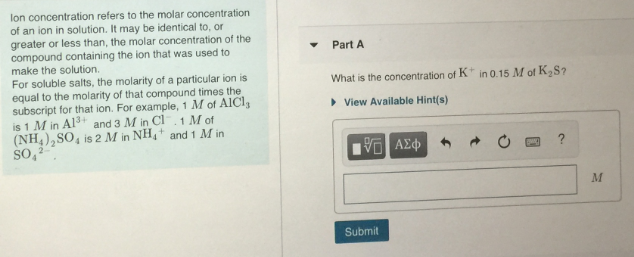# Ion concentration refers to the molar concentration of an ion in solution. It may be identical to, or greater or less than, the molar concentration of the compound containing the ion that was used to make the solution. For soluble salts, the molarity of a particular ion is equal to the molarity of the compound times the subscript for that ion. For example, 1M of AlCl3 is 1M in Al3+ and 3M in Cl-. 1M of (NH4)2SO4 is 2M in NH4+ and 1M in SO42-. What is the concentration of K+ in 0.15M of K2S?